# HTML5 Tutorial: Draw Circles and Arcs on Canvas

Simeon Visser is a writer who enjoys covering topics such as technology and travel.Drawing circles and arcs in HTML5 can be quite a challenge.Christian Holzinger/Unsplash

## Let's Begin

In HTML5, we can draw the most beautiful shapes by including circles and arcs in our drawings. In this HTML5 tutorial, I will show you how to draw a circle or an arc on an HTML5 canvas. You will see that they are technically not that different from each other. This tutorial has many examples as it is not always straightforward how to draw those circles and arcs the way you want them.

Be sure to read my tutorial on the basics of drawing on the canvas first before continuing with this tutorial. This will explain what a drawing context is and how to use it.

## Basic HTML5 webpage

We start this tutorial with a basic empty HTML5 webpage. We have also added some code to see the drawing context that we need to draw later on. You won't see anything when viewing this webpage in a browser. It is a valid HTML5 webpage, however, and we will extend it in the rest of this tutorial.

## The Arc Method of the Drawing Context

In the code above, we have created a drawing context ctx. Both drawing a circle and drawing an arc are done using the same method arc of the drawing context ctx. This can be done by calling arc(x, y, radius, startAngle, endAngle, counterClockwise) with values filled in for each of these arguments.

The x and y arguments are the x-coordinate and y-coordinate of the arc. This is the center of the arc or circle that you are drawing.
The radius argument is the radius of the circle along which the arc is drawn.
The startAngle and endAngle arguments are the angles where the arc begins and ends in radians.
The counterClockwise argument is a boolean value that specifies whether you're drawing in a counter-clockwise direction or not. By default, arcs are drawn clockwise, but if you have true as an argument here, then the arc will be drawn counter-clockwise. We will use the value false as we will draw clockwise.

The most important things you need to know about the start and end angles are the following:

1. The values of these angles go from 0 to 2 * Math.PI.
2. A start angle of 0 means starting to draw from the 3 o'clock position of a clock.
3. An end angle of 2 * Math.PI means drawing until the 3 o'clock position of a clock.
4. All start and end angles in between are measured by going clockwise from the start towards the end (so from 3 o'clock to 4 o'clock all the way back to the 3 o'clock position again). If you have set counterClockwise to true then this goes counter-clockwise.

This means that if you want to draw a circle, you need to start at 0 and end at 2 * Math.PI because you want to start your arc at the 3 o'clock position, and you want to draw the arc all the way back to that 3 o'clock position (2 * Math.PI). This makes a full circle. If you want to draw any arc that is not a full circle, you need to pick the start and end angles yourself.

In particular, note that you do not specify the length of the arc but only the start and end angles in a predefined system (with 0 at the 3 o'clock position of a circle).

0

0

90

0.5 * Math.PI

180

Math.PI

270

1.5 * Math.PI

360

2 * Math.PI

## How Are the Start and End Angle of an Arc Measured?

The start and end angle of the arc method are measured in radians. Let me quickly explain what that means: a full circle has 360 degrees which is the same as 2 times the mathematical constant pi. In JavaScript the mathematical constant pi is expressed as Math.PI and I will refer to pi like that in the rest of this tutorial.

In the table, you'll see the most common start and end angles for your circles and arcs. Look at this table whenever you're confused about what you're exactly drawing and what the angles need to be.

You should use this table if you need to convert degrees to radians in order to draw your arc.

How do you use this table?

Knowing that the arc will be drawn from the 3 o'clock position, determine how far away in degrees the arc will start or stop and look up the starting angle in radians. For example, if you start drawing at the 6 o'clock position, that's 90 degrees away from the starting point, and therefore the start angle is 0.5 * Math.PI.

Scroll to Continue

## Read More From Owlcation

Similarly, if you end drawing the arc at the 12 o'clock position, then you need to use 1.5 * Math.PI because we are now 270 degrees away from the starting point.

## How to Draw an Arc or Circle in HTML5

In the sections above, I explained the values that you need to specify an arc, such as its location and what the angles are. I'm now going to explain how to actually draw the arc so that it becomes visible on your canvas.

As discussed in a previous tutorial, you can either stroke or fill your arc on the canvas. Here's an example of what drawing a circle could look like:

## Examples of Drawing a Circle in HTML5

As explained above, we need a start angle of 0 and an end angle of 2 * Math.PI. We cannot vary these values, so the only arguments that can vary are the coordinates, the radius, and whether or not the circle is drawn counter-clockwise or not.

We're talking about a circle here so the last argument also doesn't matter (it can be either false or true) because you need to draw the whole arc (circle) anyway. The only arguments that matter are therefore the coordinates and the radius.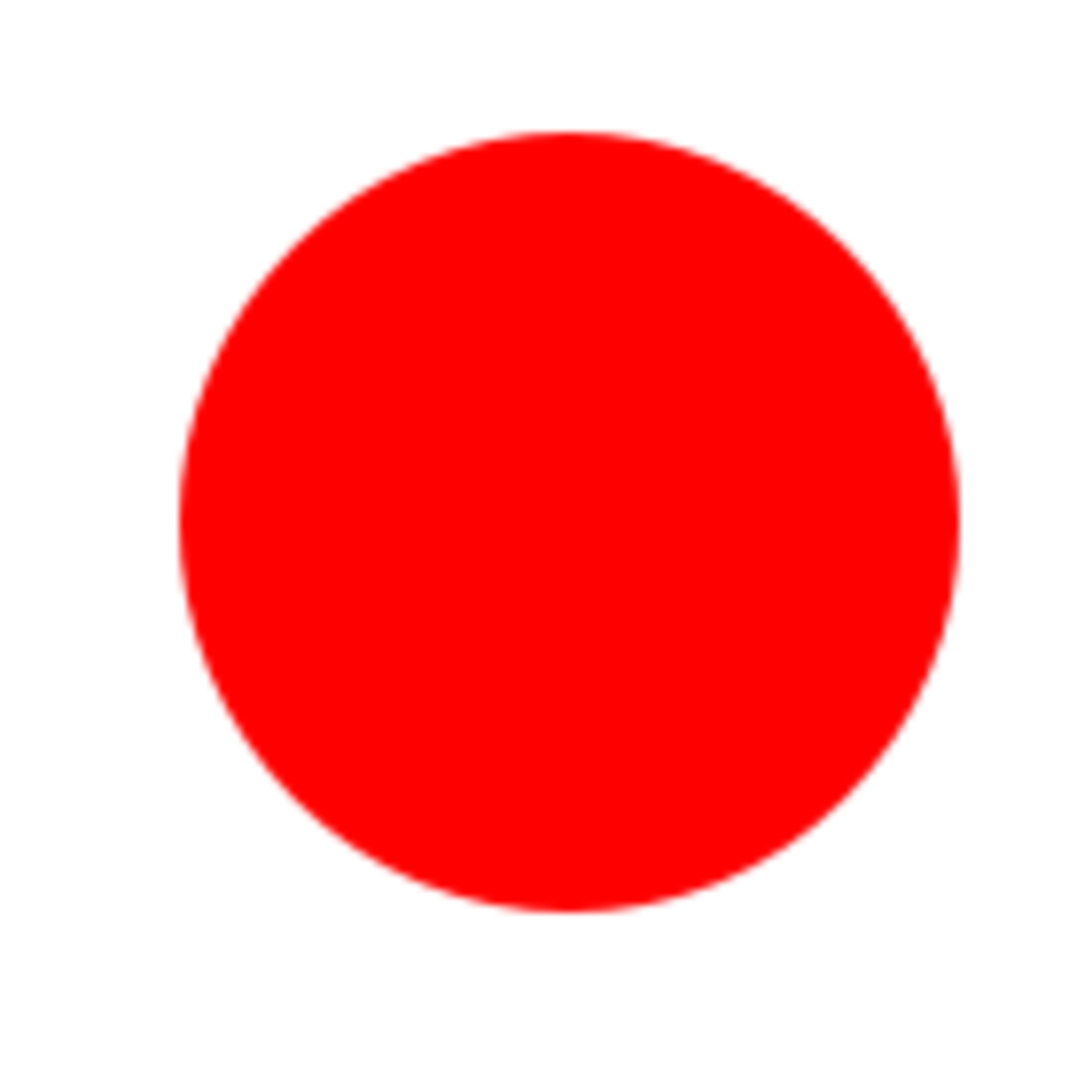A red circle centered at coordinate (100, 100) with a radius of 50.

Here are some examples of drawing a circle in HTML5:

A red circle centered at coordinate (100, 100) with a radius of 50.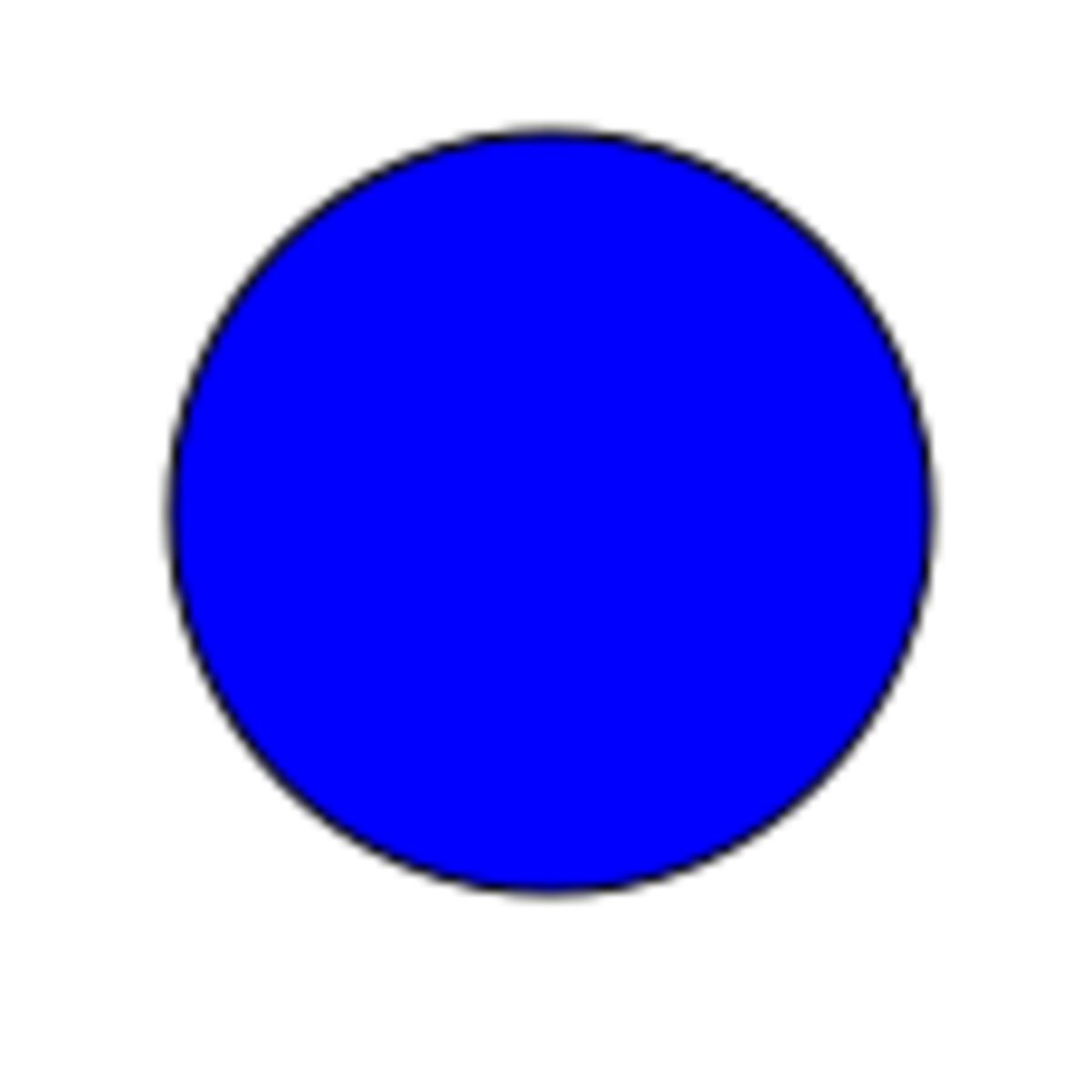A blue circle with a black border centered at (80, 60) with a radius of 40.

## Examples of Drawing an Arc in HTML5

We can now choose the start and end angles of the arcs. Remember to look at the table above with degrees and radians if you are confused. For convenience, all the following examples will have an arc centered at (100, 100) and a radius of 50, as these values don't really matter to understand how to draw an arc.

Here are some examples of drawing an arc in HTML5: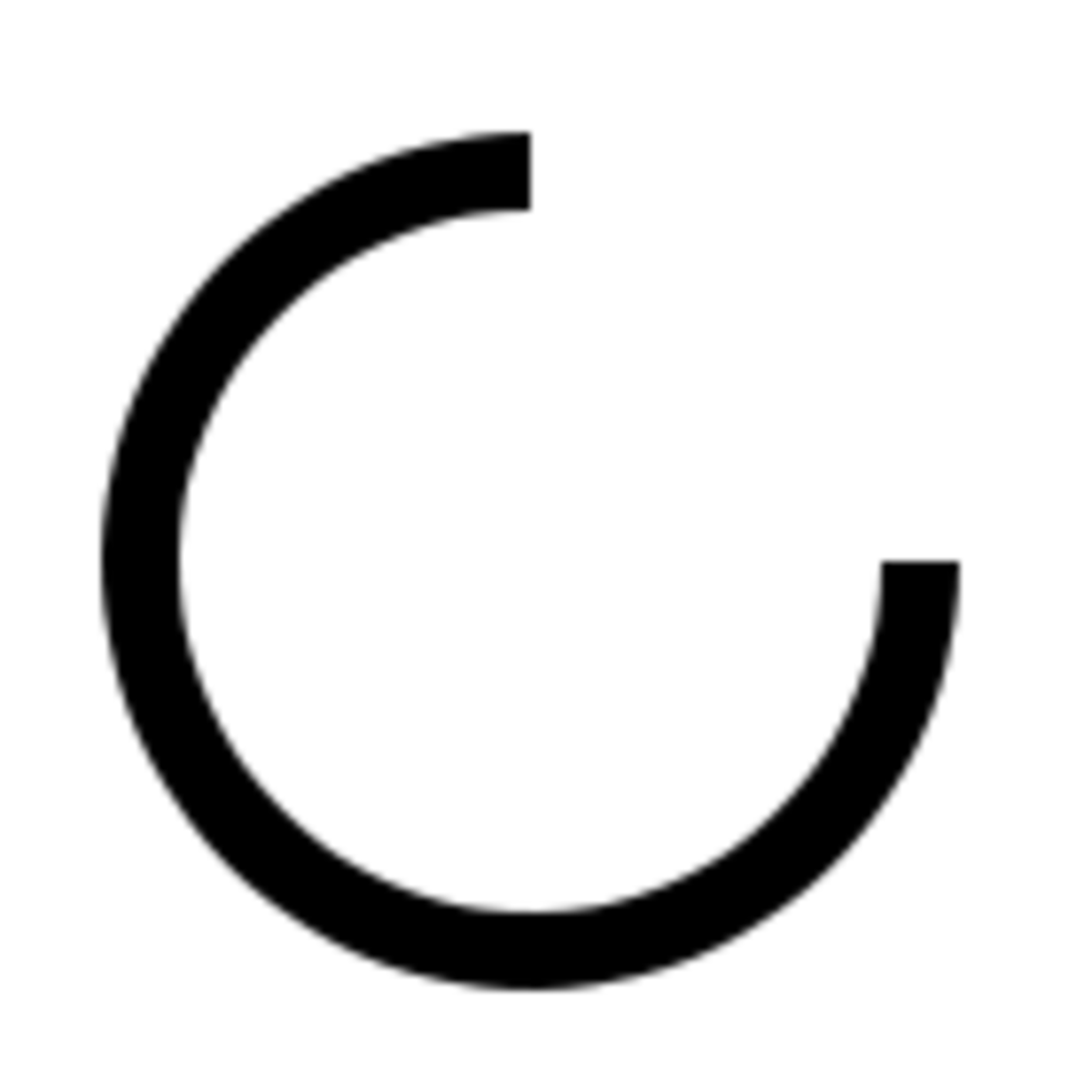A clockwise arc starting from the 3 o'clock position (0) to the 12 o'clock position (1.5 * Math.PI). This is an arc of 270 degrees.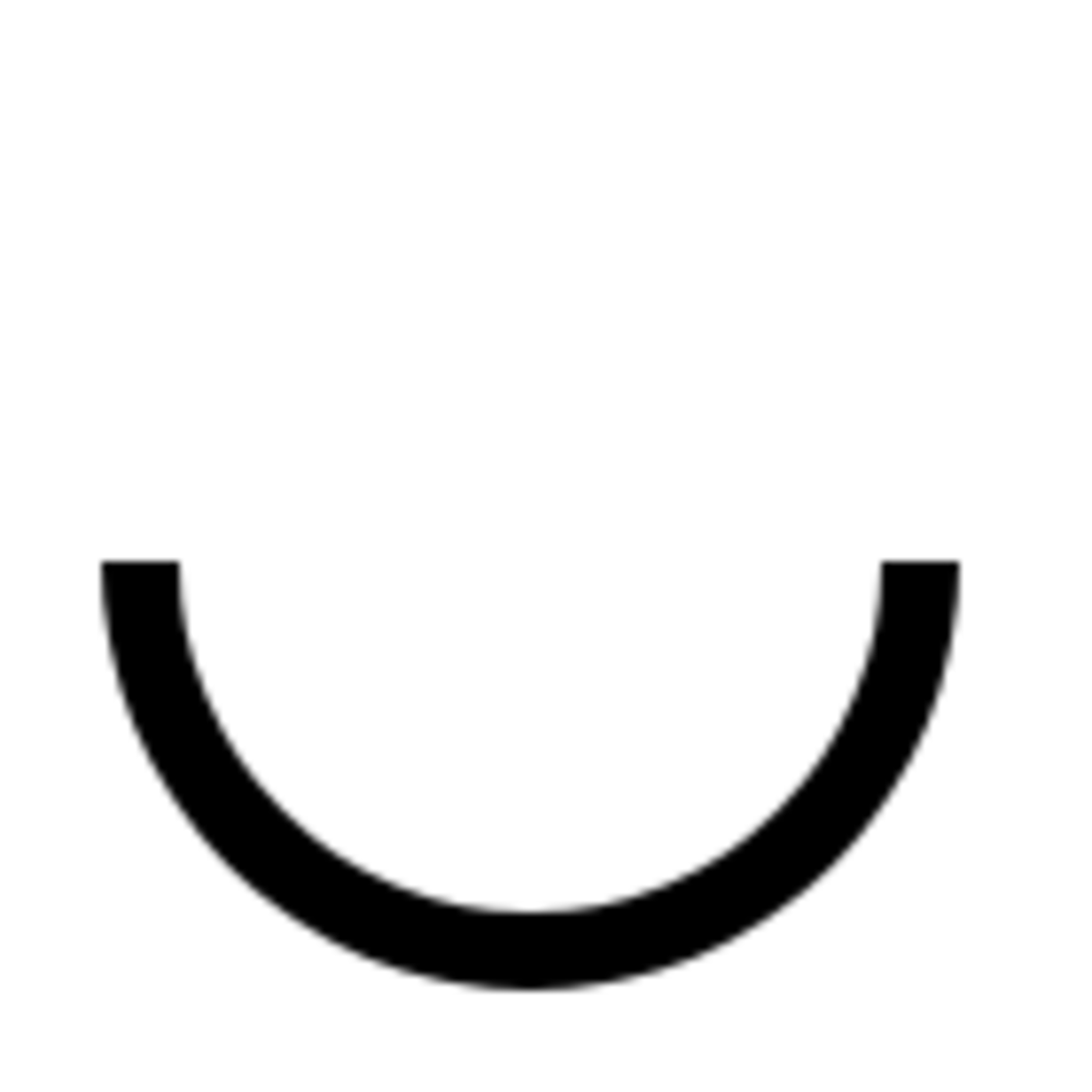A clockwise arc starting from the 3 o'clock position (0) to the 9 o'clock position (Math.PI). This is an arc of 180 degrees and the bottom half of a circle.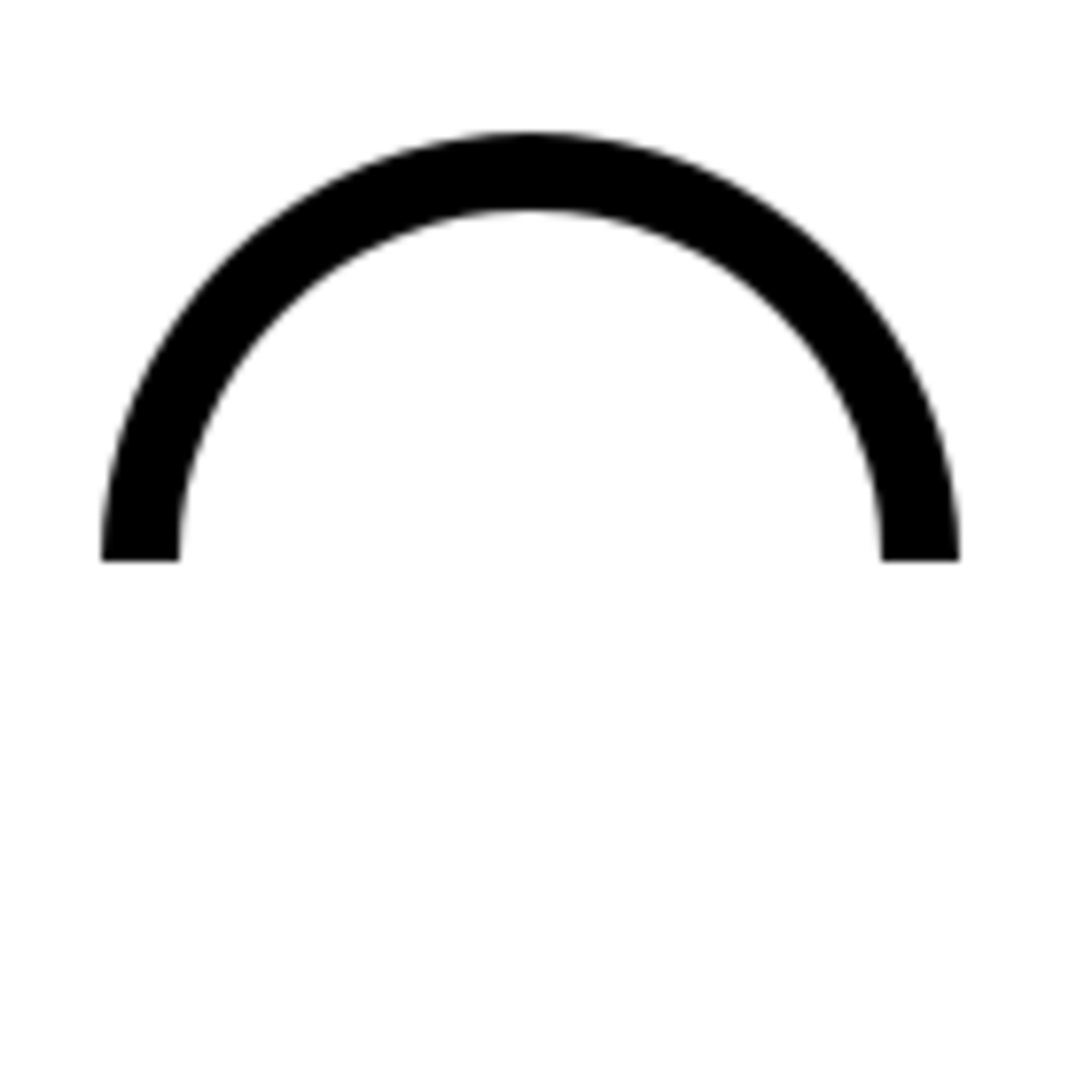A clockwise arc starting from the 9 o'clock position (Math.PI) to the 3 o'clock position (2 * Math.PI). This is an arc of 180 degrees and the top half of a circle.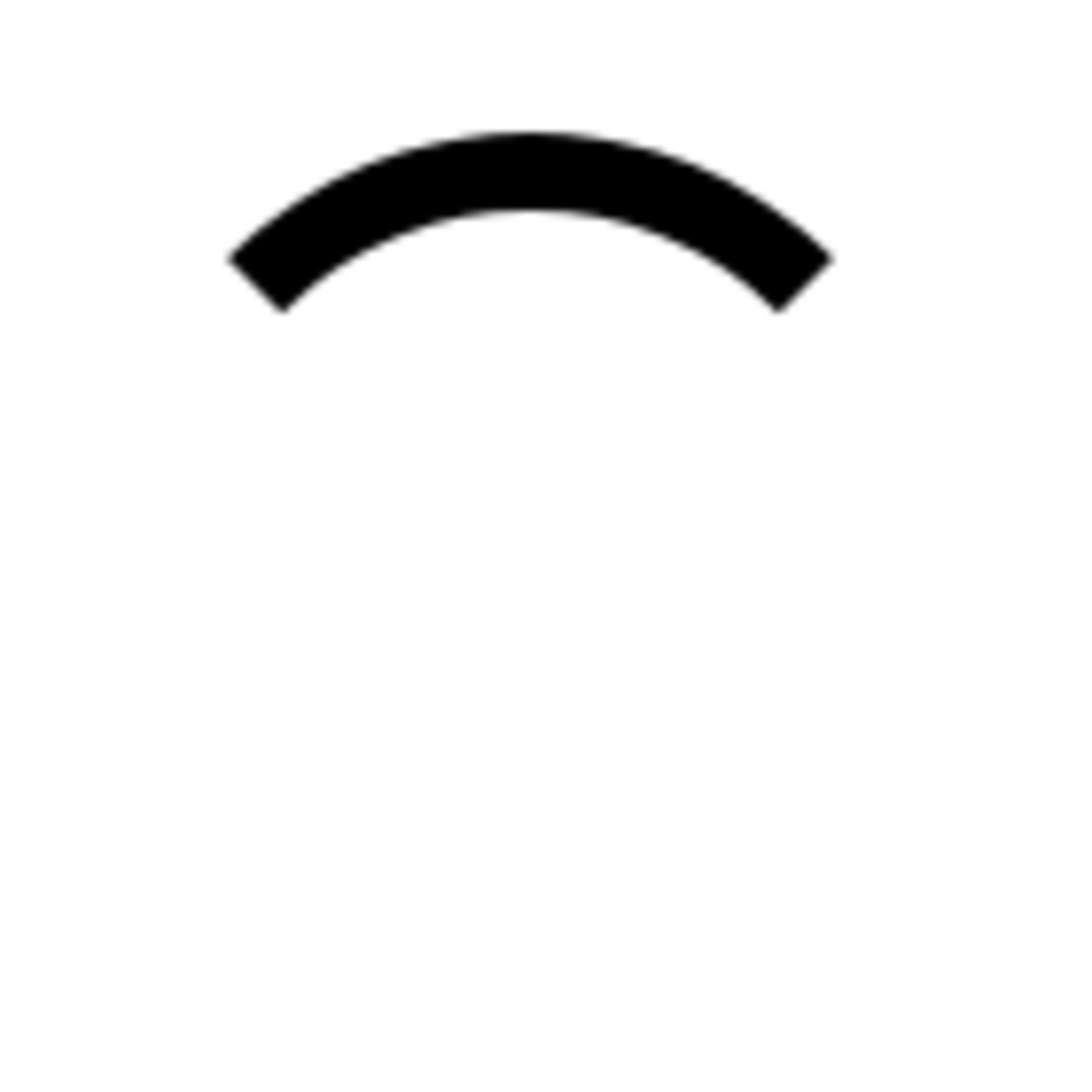A clockwise arc starting from start angle 1.25 * Math.PI to end angle 1.75 * Math.PI. This is an arc of 90 degrees.

## What if the Start Angle Is Higher Than the End Angle?

No worries, it will still draw an arc. Have a look at this example:

Can you figure out why it still works?

## Example of Circles and Arcs: Pac-Man in HTML5

As a final example of drawing circles and arcs in HTML5, have a look at the following example of Pac-man in HTML5!

## Another Great HTML5 Tutorial!

Mudasir Malik on July 16, 2013:

that is quite great to read your tutorial, it helped me a lot and hope that this will keep doing help the freshers.

Vishal Jaiswal on May 11, 2013:

I Like It and thanks for HELP me.

jessy on July 12, 2012:

is it possible to add a text in to a circle in canvas???????

sdsad on June 11, 2012:

vbulletinskins on February 28, 2012:

useful tutorial. Thanks for sharing it!

InduswebiTech from Rama Road, Kirti Nagar, New Delhi, India on January 06, 2012:

wow .... really usefull information thanks for sharing it with us....

www.induswebi.com

simeonvisser (author) on August 14, 2011:

I'm glad it was useful to you :)

cweed on August 09, 2011:

Hey I've been searching for a tutorial to explain arc() and specifically its arguments in simple plain English. There aren't many out there - yours is amazing though, thank you!

brennawelker on July 05, 2011:

Wonderful tutorial! You had a great job on this.

simeonvisser (author) on January 21, 2011:

@dalogi: No problem, I had to play a bit with it myself as well before it became clear. I'm @simeonvisser on Twitter, I've started to follow you :)

dalogi on January 21, 2011:

I've looked *everywhere* for a simple explanation into radians, and been confused when trying to apply them to HTML5 charts. Until I came to this page. Thank you so much Simon. Truly awesome tutorial! Keep up the good work and would be great to hook up on Twitter to stay up to date with your offereings. I'm @dalogi btw ;)

simeonvisser (author) on December 29, 2010:

@life.object: Thanks!

@thisisoli: I know. I'm using the latest versions of several browsers but many HTML5 features have not even been implemented yet. I intend to write more tutorials when I can play around with these new HTML5 features. I should also look into CSS3 more. HTML5 and CSS3 make a great combination.

thisisoli from Austin, Texas (From York, England!) on December 29, 2010:

Great hub :) I have been playing around with CSS3, but HTML5 is definitely calling!

life.object from Lahore, Pakistan on December 22, 2010:

Amazing Tutorial

simeonvisser (author) on December 18, 2010:

Thanks! :)

psychicdog.net on December 18, 2010:

Awesome tutorial. Thanks for providing this great info simonvisser